# 6.1 Reflection and transmission of waves

Up to this point we have largely neglected one of the most important features of the arterial system - the complexity of the arterial tree with its myriad bifurcations and frequent anastomoses. These anatomical variations in the arteries mean that the waves propagating along them are continuously altering to the new conditions that they encounter.

Any discontinuity in the properties of the artery will cause the wavefronts to produce reflected and transmitted waves according to the type of discontinuity. There are many types of discontinuities in the arterial system; changes in area, local changes in the elastic properties of the arterial wall, bifurcations, etc. We will mainly consider two types of discontinuity: changes in properties in single arteries and bifurcations.

Before getting into the mathematical details, here is are sketches of what would happen in the simple wave example if the tube either narrowed or widened at some point.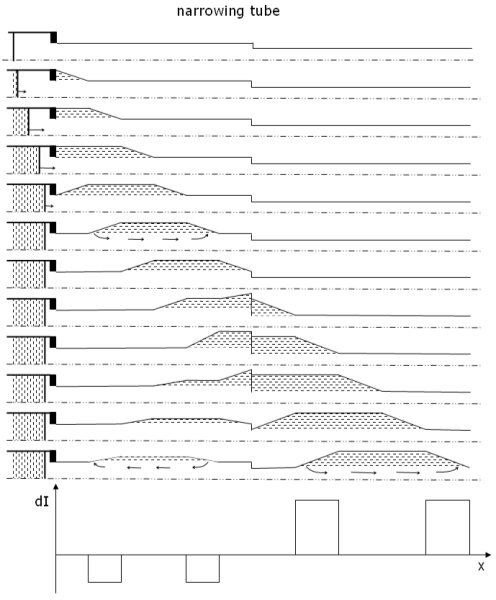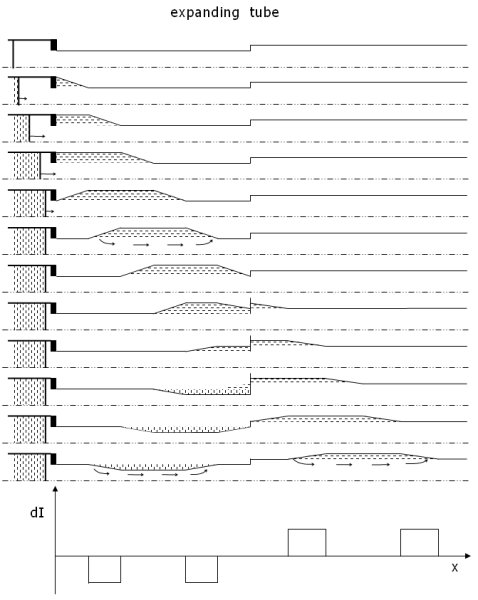Simple example of a wave in a tube that narrows The reflection coefficient is positive so that the leading forward compression wavefront reflects as a backward compression wave and the trailing expansion wave reflects as an expansion wave. The transmitted wave must match the pressure produced by the incident and reflected waves at the discontinuity in area giving a wave of similar form but with an increased amplitude. Simple example of a wave in a tube that widens The reflection coefficient is negative so that the leading forward compression wavefront reflects as a backward expansion wave and the trailing expansion wave reflects as a compression wave. The transmitted wave is similar in form to the incident wave with a reduced amplitude because of the negative reflection. Note the direction of 'circulation' in the different waves.

Here are animated versions of the simple wave example in a tube that narrows, with an decreased distensibility (and hence a faster wave speed) and in a tube that expands, with a increased compliance (and hence a slower wave speed). These figures are simply sketches and do not show all of the detail of the interaction of the wave with the discontinuity.

For a narrowing vessel with a reflection coefficient R=+0.5, the reflected wave is a compression wave that produces flow in the backward direction.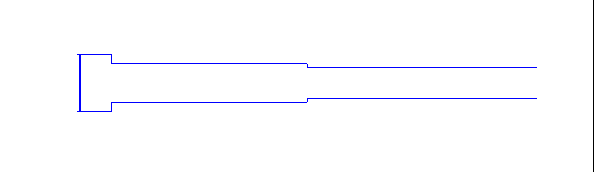For an expanding vessel with a reflection coefficient R=-0.5, the reflected wave is an expansion wave that produces flow in the forward direction.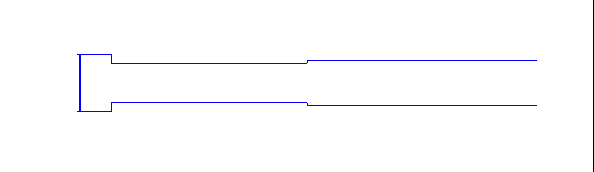### Reflections in a single vessel

The mathematical details involved in deriving the exact nature of the reflected and transmitted waves is rather complex but the results are straightforward and will be outlined here. The conservation of mass and energy at a discontinuity in an elastic vessel require that an incident wave with a pressure change ΔP must generate a reflected wave with pressure change δP that is given by a reflection coefficient R = δP/ΔP. This definition is familiar from many branches of wave mechanics when it is remembered that pressure has the units of energy per unit volume. The value of the reflection coefficient depends upon the area A and wave speed c upstream 0 and downstream 1 of the discontinuity. For arteries where the velocity is generally much lower than the wave speed the equation for R is valid {mathematical details} so that
 R  = (A0/c0) - (A1/c1)(A0/c0) + (A1/c1)

This expression varies with the ratios of areas and wave speeds upstream and downstream. There are two simple limits:

1) closed tube, A1 = 0 for which R = 1
2) open tube, A1 = ∞ for which R = -1

All other cases will lie between these two limits. The transmission coefficient T is simply related to the reflection coefficient

T = 1 + R

Physically these limits mean that a wavefront encountering a closed end will be reflected with exactly the same pressure change. Remembering the water water hammer equations

dP± = ± ρc dU±

this means that the change velocity across the reflected wavefront will be opposite that of the incident wavefront.

### Reflections in a bifurcation

If we consider a bifurcation where the parent vessel is 0 and the daughter vessels are 1 and 2, the conservation equations can be solved for the reflection and transmission coefficients. The results for m << 1 are {mathematical details}

 R  = (A0/c0) - (A1/c1) - (A2/c2)(A0/c0) + (A1/c1) + (A2/c2)

This relationship depends on both the areas and the wave speeds (which depend on the distensibilities of the vessels). If all of these data are know, the reflection coefficient can be easily found. For a general discussion, it is useful to make an assumption about the variation of the wave speed with vessel size so that R can be expressed as a function of the areas of the vessels. A reasonable assumption is that c ~ A-1/4. This follows from the Moens-Korteweg equation for the wave speed in thin-walled, uniform tubes if it is assumed that the product of the elastic modulus and the thickness of the vessel wall are constant. Since arteries are not thin-walled and their wall composition and structure changes from vessel to vessel, this assumption is only an approximation. However, it does fit experimental data for the wave speed in arteries of different diameters reasonably well.

Define α as the daughters to parent area ratio

α = (A1 + A2)/A0

and γ as the daughter symmetry ratio (we assume without loss of generality that A2 < A1)

γ = A2/A1

The extreme values γ = 0 corresponds to a single vessel with no branches and γ = 1 corresponds to a symmetrical bifurcation. The reflection coefficient can now be expressed in terms of these two area ratios
 R  = (1 - (α/(1+γ))5/4(1 + γ5/4) (1 + (α/(1+γ))5/4(1 + γ5/4)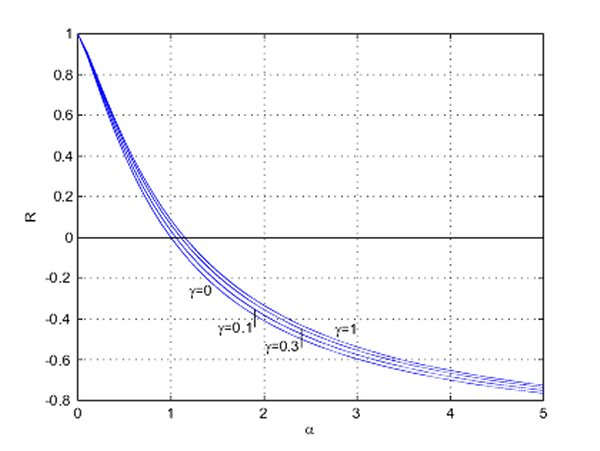We see for symmetrical bifurcations, γ = 1, that R = 0 for an area ratio α ~ 1.15. For α less than this the bifurcation acts like a partially closed tube and R is positive. For α greater than this value the bifurcation acts more like an open tube and R is negative. Reflection coefficient as a function of area ratio for different symmetry ratios

For a symmetrical bifurcation that is well-matched in the forward direction, the area ratio for a wave travelling backwards in one of the daughter vessels is approximately &alpha = 2.7. The reflection coefficient for this backward wave is approximately R = -0.5 which means that approximately half of the energy of the backward wave will be reflected back in the forward direction and that this wave will be of the opposite type as the incident wave (i.e. a compression wavefront will be reflected as an expansion wavefront and an expansion wavefront will be reflected as a compression wavefront.

This is reasonable physically because the backward wave approaching the bifurcation in one of the daughter vessels (now the parent vessel) will see a bifurcation consisting of its twin vessel and the parent vessel with a net area much larger than its own. The bifurcation will therefore act more like an open-end tube and generate a negative reflection coefficient.